Operator Calculus:   Linear Operator Rules

Does Mathematica  apply the Fourier Transform/Derivative Rule Automagically?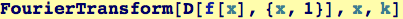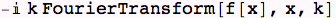It does as of version 5.0! .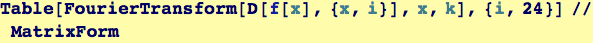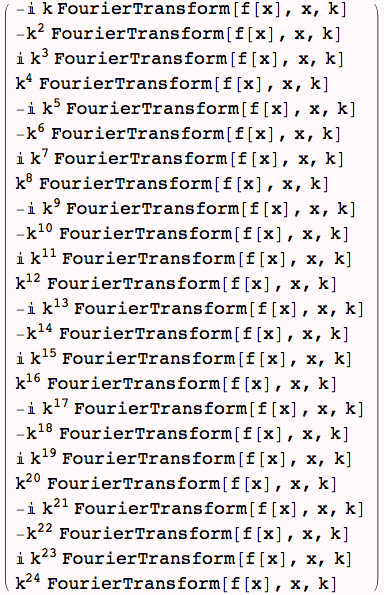Does  Mathematica apply the rules according to the Fourier Transform being a linear operator?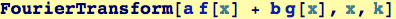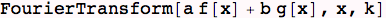Apparently not--so we make some rules that can be applied. It may be instructive to see how to do this.

Two rules are defined so as to factor out non-dependent variables and apply the distribution rules for linear operators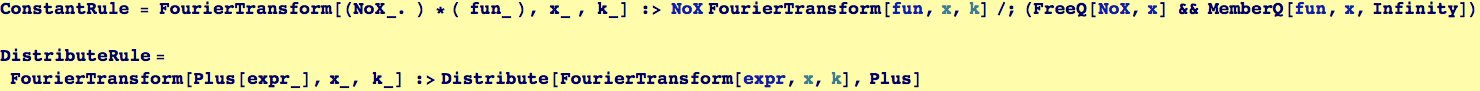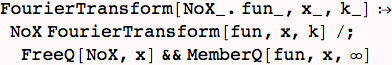Try them out: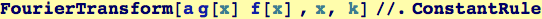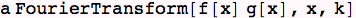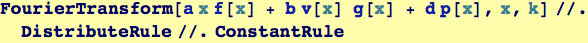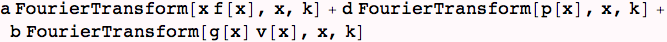Created by Wolfram Mathematica 6.0  (26 November 2007)